# How to Convert Percentages to Fractions

How to Convert Percentages to Fractions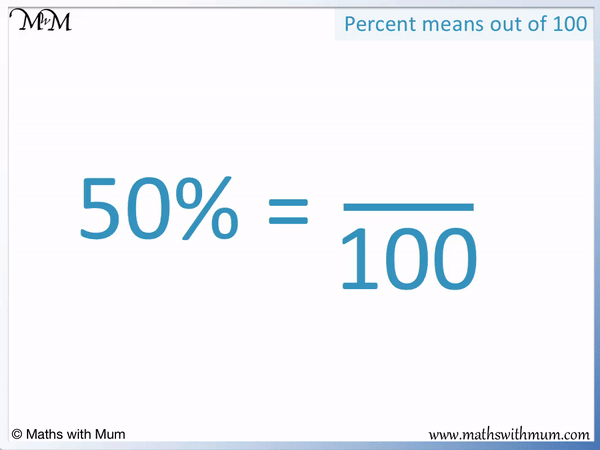• In the word percent, per means ‘out of’ and cent means ‘100’.
• To convert a percent into a fraction, we write the number out of 100.
• 50% means 50 out of 100 or written as a fraction,   50 / 100   .
• We simply remove the percentage sign and write the number as a fraction out of 100.
• We can then simply the fraction afterwards if necessary.
•   50 / 100   is   1 / 2   because 50 is half of 100.
Remove the % sign and write the number as a fraction out of 100.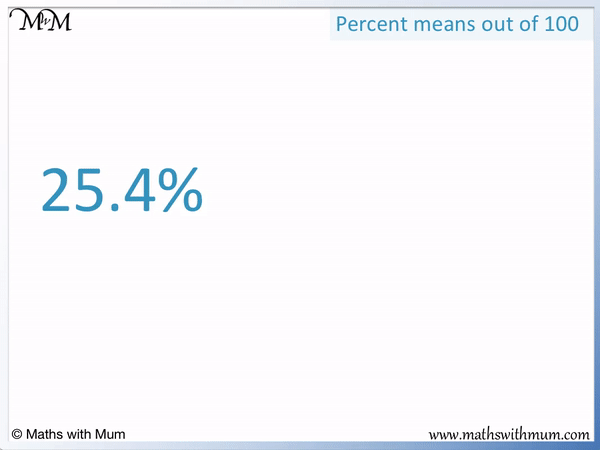• To convert a percentage to a fraction, remove the % sign and write the number as a fraction out of 100.
• 25.4% is   25.4 / 100  .
• We don’t normally include decimals in fractions, so we can multiply the numerator and denominator both by 10.
•   25.4 / 100   is an equivalent fraction to   254 / 1000  .
• We can now simplify the fraction by dividing the numerator and denominator by 2.
•   254 / 1000   can be simpified to   127 / 500  .
• 25.4% can be written as a fraction in simplified form as   127 / 500Supporting Lessons

#### Converting a Percent with a Decimal to a Fraction# Writing Percentages as Fractions

## How to Convert a Percentage to a Fraction

To convert a percentage to a fraction, use these steps:

1. Remove the percentage sign and write the remaining number as a fraction out of 100.
2. For each decimal place in this number, multiply the numerator and denominator of the fraction by 10.
3. Simplify the fraction if possible.

Here is an example of converting a percentage to a fraction in simplest form. We have 50%.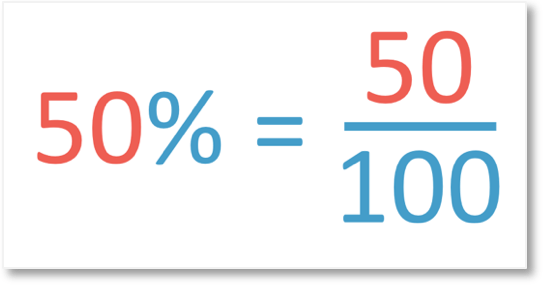Step 1: Remove the percentage sign and write the remaining number as a fraction out of 100.

We remove the % sign and write 50 out of 100.

Step 2: For each decimal place in this number, multiply the numerator and denominator of the fraction by 10.

We do not need to change the fraction because it does not have any decimal places. 50 does not have any decimal parts.

Step 3:Simplify the fraction if possible.We can simplify the fraction   50 / 100   to   1 / 50  . We can notice that 50 is one half of 100 or simply divide the numerator on top and the denominator on the bottom both by 50.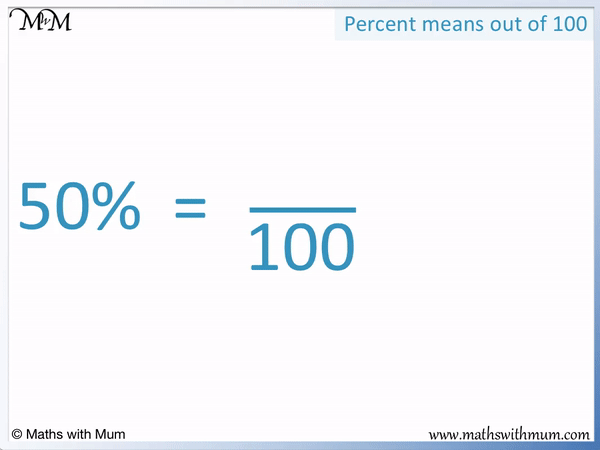Here are a series of examples of percentages written as fractions.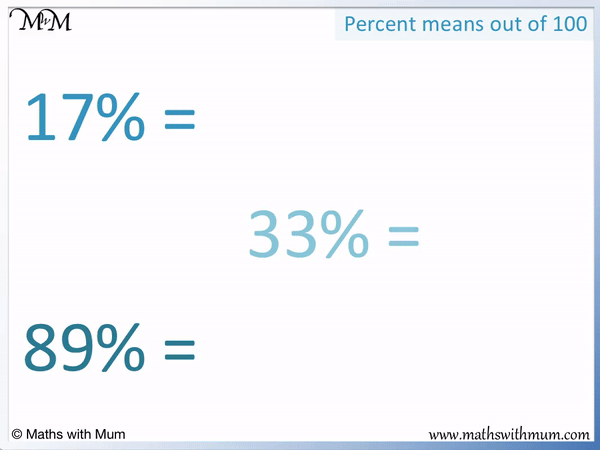In all of these examples, the number can simply be written as a fraction out of 100 in one step.

17% can be written as   17 / 100  .

33% can be written as   33 / 100  .

89% can be written as   89 / 100  .

There is no need to simplify these fractions because there is not a number that divides exactly into each of 17 and 100, 33 and 100 or 89 and 100.

Percentages are written out of 100 because the word derives from the Latin words ‘Per Centum’. Per means ‘out of’ and cent means ‘one hundred’. Put together, percent means ‘out of 100’. The percentage symbol % is a shorter way to write ‘out of 100’.

Whilst all percentages can be written as a fraction out of 100, not every percentage will always be out of 100. If the fraction contains a decimal, it will be changed to an equivalent fraction that is not out of 100. Also, if a fraction is simplified, it will no longer be out of 100.

## How to Write a Percent as a Fraction in its Simplest Form

To write a percent as a fraction in its simplest form, follow these steps:

1. Remove the percentage sign.
2. Write the remaining number as the numerator above a denominator of 100.
3. If the numerator and denominator can both be divided exactly by the same amount without remainder then divide them by this amount.
4. Repeat step 3 as many times as necessary there is no number that divides exactly into both the numerator and denominator.

For example, here is the percentage 15%.

Following step 1, we remove the percentage sign and write 15 out of 100,   15 / 100  .The numerator and denominator end in 5 and 0 respectively. Therefore both 15 and 100 are both in the 5 times table. We can divide them both by 5.15 / 100   can be written as   3 / 20  . This is because 15 ÷ 5 = 3 and 100 ÷ 5 = 20. We must divide the numerator and denominator by the same amount to keep the fractions equivalent, or the same size.

We cannot divide both 3 and 4 by another number without decimals forming in our fraction. We do not write decimals in fractions and so, we leave 15% as   3 / 20  .Here is another example of writing a percentage as a fraction in its simplest form.

We have 75%. 75 percent means 75 out of 100. We write this as   75 / 100  .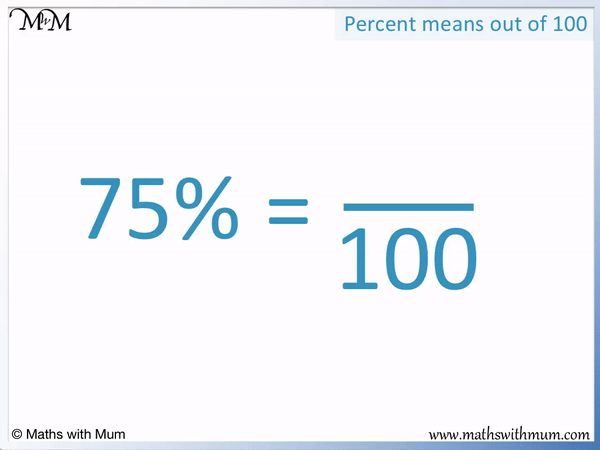We can then simplify this fraction. We know that the fraction can be simplified because 75 and 100 end in 5 and 0 respectively. Numbers that end in 5 and 0 can be divided by 5.We can divide the top and bottom by 5 to get   15 / 20  , however, we can simplify this further by dividing by 5 one more time.

We can fully simplify   15 / 20   to   3 / 4  .

We can see that   75 / 100   can be simplified to   3 / 4   in one go by dividing the numerator and denominator both by 25.75 ÷ 25 = 3

100 ÷ 25 = 4

Here is another example of writing a percentage as a fraction in its simplest terms.

We have 4% and will show it as a fraction by writing 4 out of 100.We can then see that both 4 and 100 are even as they end in an even number. This means that both the numerator and the denominator can be divided by 2.

4 ÷ 2 = 2

100 ÷ 2 = 50

4 / 100   can be written as   2 / 50  . Both 2 and 50 also end in even numbers and so, can be halved once more.

2 ÷ 2 = 1

50 ÷ 2 = 254% is   4 / 100   which can be fully simplified to   1 / 25  .

If you spot that 4 and 100 are both divisible by 4, then this simplification can be done in one step.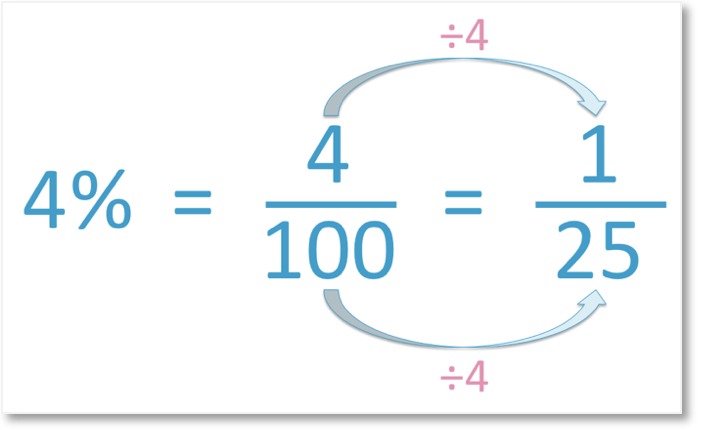4 ÷ 4 = 1

100 ÷ 4 = 25

## How to Write a Percent with a Decimal as a Fraction

To write a percent with a decimal as a fraction, follow these steps:

1. Remove the percentage sign and write the remaining number as a fraction out of 100.
2. For every digit after the decimal point, multiply the numerator and denominator by 10.
3. Simplify the fraction if possible.

For example, here is 25.4%.Step 1: Remove the percentage sign and write the remaining number as a fraction out of 100.

We have 25.4 out of 100.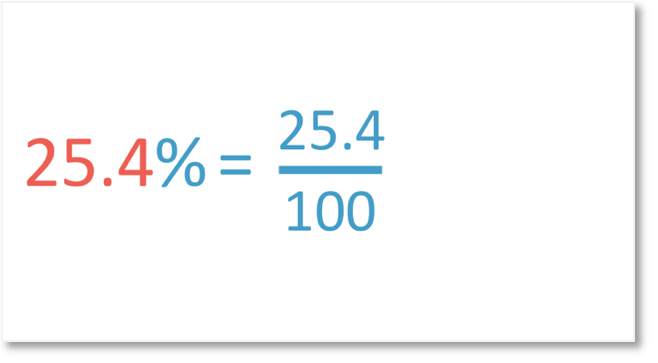Step 2: For every number after the decimal point, multiply the numerator and denominator by 10.

25.4 has one digit after the decimal point. Only the one digit of 4 comes after the decimal point.

We make 25.4 into a whole number by multiplying by 10.

25.4 × 10 = 254.

100 × 10 = 1000

We can write   25.4 / 100   as   254 / 1000  .We can then simplify   254 / 1000   by dividing the numerator and denominator by 2.

We arrive at the fully simplified answer of   127 / 500  .

In this next example we have a percentage with two decimal places.

39.04% has two digits after the decimal point, the 0 and the 4.

The first step is to write it as a fraction out of 100.Since 39.04 has two decimal places, we will need to multiply the numerator and denominator by 10 twice. This means that we need to multiply by 100.

39.04 × 100 = 3904And 100 × 100 = 10,000.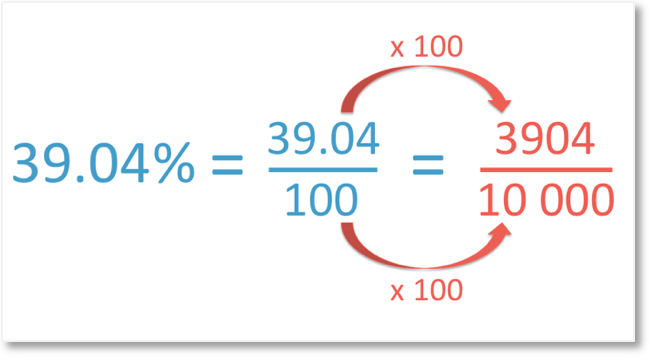39.04% can be written as a fraction as   3904 / 10,000  .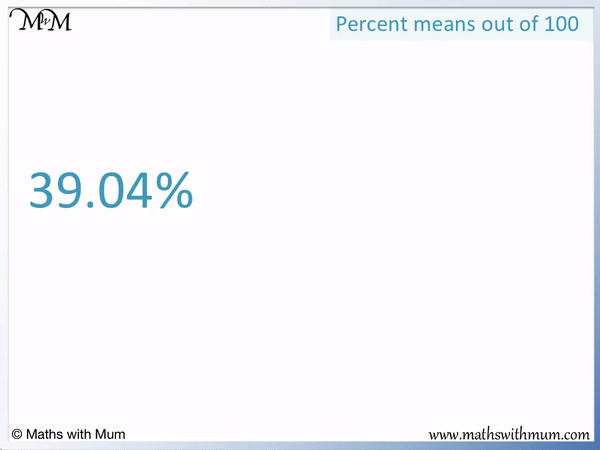We can simplify this fraction since 3904 and 10000 both end in an even number.

The numbers with some fractions can be very large, but it is possible to simplify them in chunks, particularly by looking for divisibility by 2, 3, 5 and 10.

3904 / 10000   can be simplified by dividing by 2 to get   1952 / 5000  .

1952 / 5000   can be simplified by dividing by 2 to get   976 / 2500  .

976 / 2500   can be simplified by dividing by 2 to get   488 / 1250  .

488 / 1250   can be simplified by dividing by 2 to get   244 / 625  .

So 39.04% can be written as a fraction in its simplest form as   244 / 625  .Now try our lesson on How to Write a Percentage as a Decimal where we learn how to write percentages as decimal numbers.error: Content is protected !!